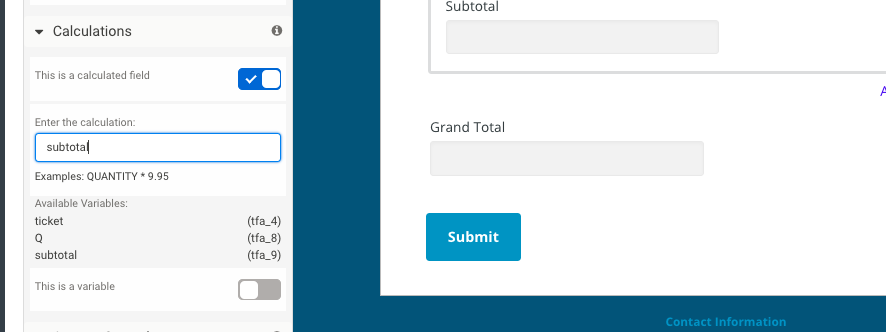# How to Use Calculations and Repeating Sections in Web Forms

#### Share

There are lots of situations where you might want to use calculations in conjunction with repeating sections. For instance, this can be especially helpful for order forms, quote forms or any other instance where you need to perform calculations based on the values given in repeating sections. For a detailed description of how to use calculations and repeatable sections, just keep reading.

## Create a Repeatable Section

First, you’ll need to create the section you’ll use in your calculation. In Form Builder, create a section of any type (in this example, we added a Fieldset), navigate to the options for that section, select “Repeatable Option” and click the button labeled “This is a repeatable section.” If you like, you can change the text for the repeat link by editing it directly in the form builder.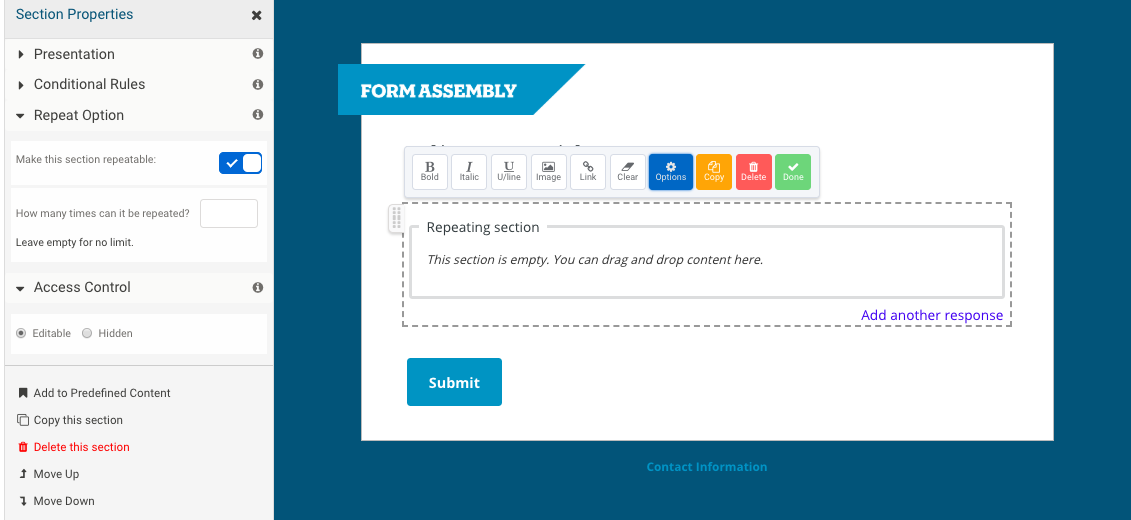## Add Questions to the Section

Next, add questions to the section. For this example, we’ll be calculating the cost of purchasing a set of tickets, including tickets of different values. First, we’ve created a drop-down menu question for the user to select the type of tickets she would like. Next, we set three different price levels for tickets.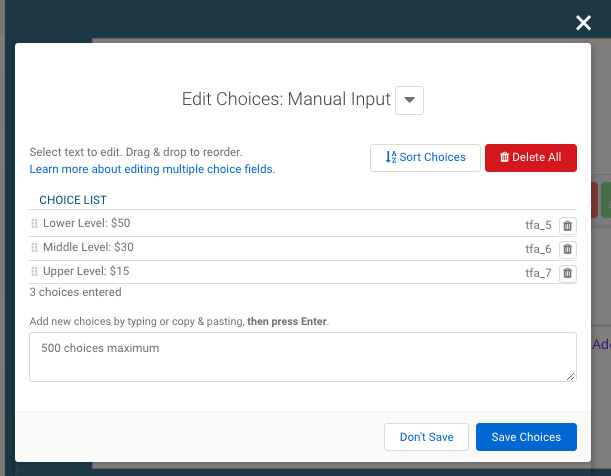Once the choices are set, click on the Options button for that question and open up Calculations, which you’ll find under the Advanced Features. Once you click the button to make the question a variable, you will be able to set values for each of the choices. You can see below that we set the value equal to the dollar amount of each ticket. The value you set here will be used in the calculation for the total price. This is also where you can set a name for your variable so you can use it later on in formulas. (We kept it simple and named it “ticket.”)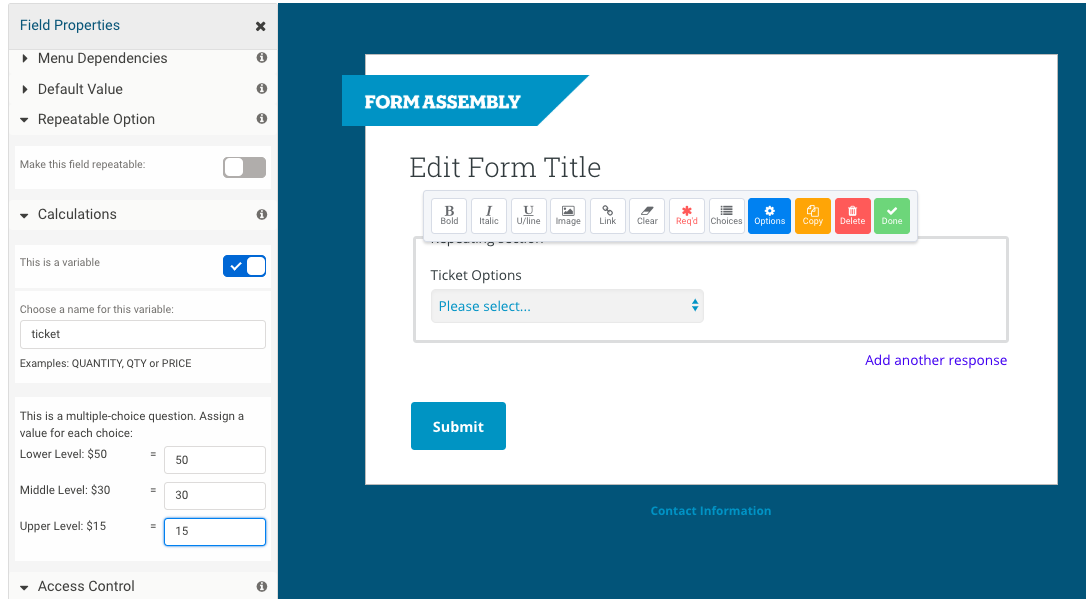Next, add a Quantity question so the user can say how many tickets she would like. As with the ticket type, be sure to open the Options, select Calculations from the advanced options, mark the field as a variable and name it. (We named our variable “Q.”)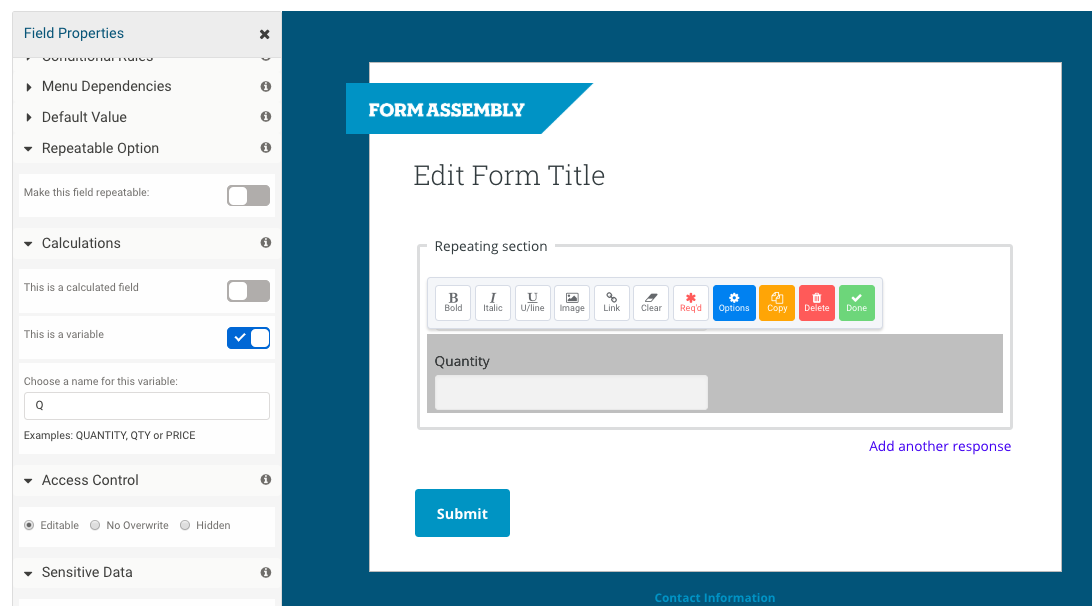Then, create the Subtotal field. This field calculates the value of the ticket and quantity fields for this particular repeatable section. We chose to make the subtotal field a hidden field.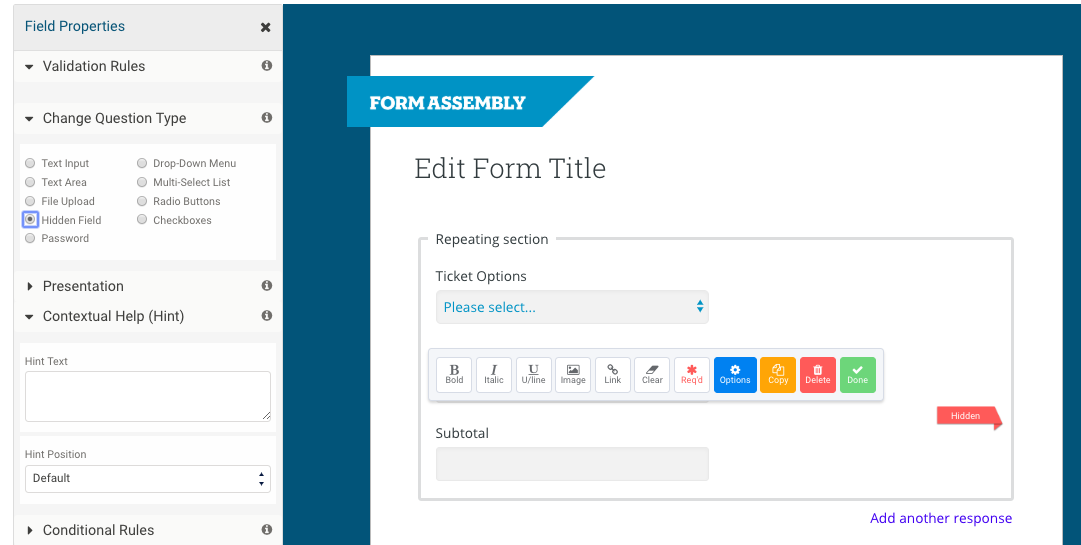In the Options sidebar under Calculations, mark the field as a calculated field, and enter the calculation you want it to perform; in this case, we will multiply ticket price times quantity. (Our formula was “ticket*Q”.) Make sure to also mark this as a variable in a calculated field, since we’ll be using it to get the grand total.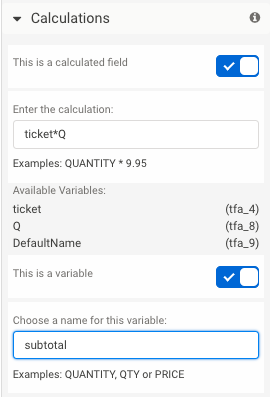## Create Grand Total Field

Finally, create the Grand Total field. Make sure to create the Grand Total field outside the repeated section. Open the Options sidebar, and mark the field as a calculated field. Simply enter the variable name of your subtotal field; the calculation will automatically add all the subtotal fields together. When you make the grand total field a calculation, it will automatically make the field appear as simple static text, instead of an input field.